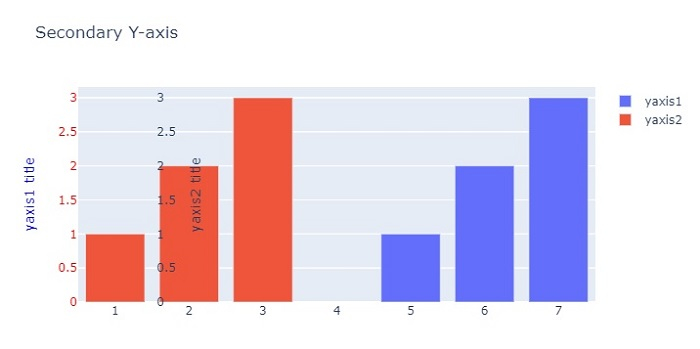# How to plot on secondary Y-Axis with Python Plotly?

Plotly is an open-source, interactive, and browser-based charting library for Python. Python users can use Plotly to generate different types of charts including scientific charts, 3D graphs, statistical charts, financial charts, etc.

In this tutorial, we will show how you can use Plotly to plot data on the secondary Y-Axis. Here we will use the plotly.graph_objects module to generate figures. It contains a lot of methods to customize the charts and render them into HTML format. We will plot two bar charts using the add_trace() method and then use the update_layout() method to set a property with dict arguments.

Follow the steps given below to plot on secondary Y-Axis.

Step 1

Import the plotly.graphs_objs module and alias as go.

import plotly.graphs_objs as go


Step 2

Create a figure using the Figure() method.

fig = go.Figure()


Step 3

Create two bar charts using the add_trace() method.

fig.add_trace(go.Bar(
x=[5,6,7],
y=[1,2,3],
name="yaxis1",
yaxis='y1'
))

x=[1,2,3],
y=[1,2,3],
name="yaxis2",
yaxis="y2"
))


Step 4

Create axis objects for the first and second Y-axis.

fig.update_layout(
xaxis=dict(domain=[0.15, 0.15]),

# create first Y-axis
yaxis=dict(
title="yaxis1 title",
titlefont=dict(color="blue"),
tickfont=dict(color="red")
),

# create second Y-axis
yaxis2=dict(
title="yaxis2 title",
overlaying="y",
side="right", position=0.15)
)


Step 5

Use the update_layout() method to set the layout and assign title text.

fig.update_layout(title_text="secondary y-axis")


## Example

Here is the complete code to plot on secondary Y-axis −

import plotly.graph_objects as go
fig = go.Figure()
x=[5, 6, 7], y=[1, 2, 3],
name="yaxis1", yaxis='y1'))
x=[1, 2, 3], y=[1, 2, 3],
name="yaxis2", yaxis="y2"))

# Create axis objects
fig.update_layout(
xaxis=dict(domain=[0.15, 0.15]),

# create first y axis
yaxis=dict(
title="yaxis1 title",
titlefont=dict(color="blue"),
tickfont=dict(color="red")
),

# Create second y axis
yaxis2=dict(
title="yaxis2 title",
overlaying="y",
side="right",
position=0.15)
)

fig.update_layout(title_text="Secondary Y-axis",
width=716, height=400)
fig.show()


## Output

It will show the following output on the browser −